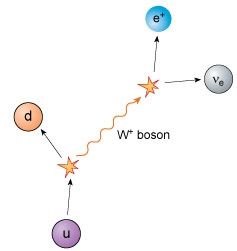# What Is W Boson Particle?

### What Is W Boson?Researchers from Collider Detector at Fermilab (CDF) Collaboration, in the US, announced that they have made a precise measurement of the mass of the W boson.

• It has been stated that this precisely determined value did not match with the estimates from the standard model of particle physics.
• The W boson was first seen in 1983 at CERN, located on the Franco-Swiss border.
• In contrast to the photon, which is massless, the W bosons are quite massive, so the weak force they mediate is very short ranged.
• European Organisation for Nuclear Research (CERN) is the world’s largest nuclear and particle physics laboratory and best known as operator of the Large Hadron Collider, which found the elusive Higgs boson in 2012.
• Unlike the photon, which is electrically neutral, the W-plus and W-minus are both massive and charged.
• By exchanging such W bosons, a neutron can change into a proton, for example:
• This is what happens in beta decay, a radioactive interaction that takes place in the sun.
• Thus, the W boson facilitates the interactions that make the sun burn and produce energy.
• The standard model of elementary particles is a theoretical construct in physics that describes particles of matter and their interaction.
• It describes the elementary particles of the world as being connected by mathematical symmetry, just as two objects are connected by bilateral (left-right) symmetry.
• These are mathematical groups generated by continuous transformations from, say, one particle to another.
• According to this model there are a finite number of fundamental particles which are represented by the characteristic “eigen” states of these groups.
• The particles predicted by the model, such as the Z boson, have been seen in experiments.
• The last to be discovered, in 2012, was the Higgs boson which gives mass to the heavy particles.